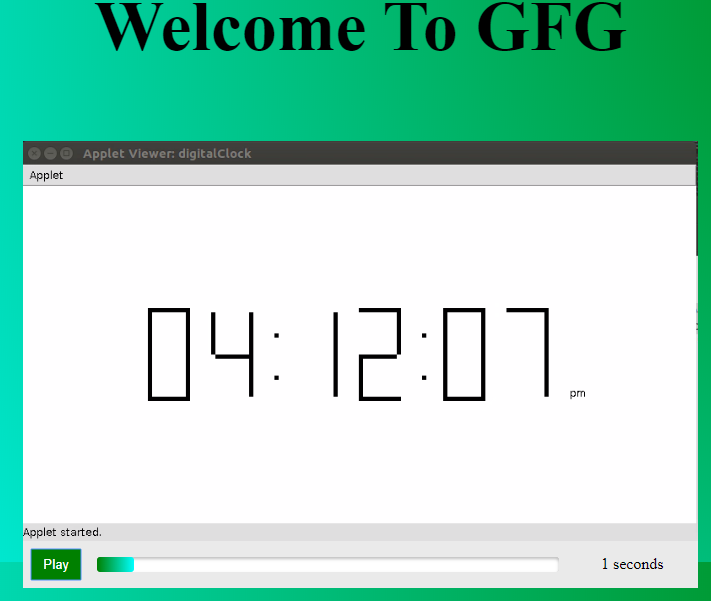# How to create a gradient Video Progress Bar?

• Last Updated : 09 Jun, 2022

Dynamic Progress Bars in videos are quite visually attractive in themselves. Here, we’ll be creating a progress bar that changes its color gradient using simple HTML, CSS, and some JavaScript. The gradient here refers to changes in color from one to another quite smoothly. Here we will be changing the gradient color from green to cyan.

Pre-built function used: Mainly we will just need the setInterval() method.

Syntax:

`setInterval(function_reference, time interval)`

Let us start by creating the HTML code:

• Example: HTML code

## html

 `` `<``html``>` `<``head``>` `<``title``>Gradient Progress Bar` `` `<``body``>` `<``h2``>Welcome To GFG` `<``figure``>` `  ``<``video` `id="video" ` `         ``src="GFG.mp4">` `    ``` `  ``<``figcaption``>` `    ``<``button` `id="play" aria-label="Play" role="button">` `        ``Play` `     ``` `    ``<``progress` `id="progress" max="100" value="0">` `            ``Progress` `    ``` `    ``` `` `` ``

The main line here is:

`<progress id="progress" max="100" value="0">Progress</progress>`

Here, the max attribute tells us that the maximum value in the video here will be 100 and the value attribute tells that we are starting from 0.

We chose this as it is quite simple to understand in terms of percentage of the video played with 100 being completely played and 0 is not started yet.

Next, we add the CSS of our choice:

• Example: CSS code

## css

 `progress[value] {` `  ``appearance: ``none``; ` `  ``border``: ``none``;     ` `  ``color``: ``green``;   ` `}`   `progress[value]::-webkit-progress-value {` `  ``background-image``: linear-gradient(` `    ``to ``right``,` `    ``#00ff00``, ``#014f01` `  ``);` `  ``transition: width ``1``s linear;` `}`

Now, begins the JavaScript coding for the progress bar. We’ll be doing this step by step so that you could understand quite easily.

Getting Progress Values from the video:

Let’s do this in steps:

• Get the current time of the video.
• Get the total duration of the video.
• Calculate the progress value using the basic math formula of percentages:  (currentTime/duration = progressValue)
• The Math.round() function will then update the results to nearest whole integers.

The basic task is done, You will have a gradient progress bar using the above steps.

Further, we’ll be adding some extra features listed below:

• Showing the current time.

Showing the current time:

This is quite easy to do! We can just count the seconds as the video plays and show them in a timer next to the progress bar.

• Example:

Add a timer label in the HTML file:

## html

 `<``figure``>` `  ``<``video` `controls id="video" ` `         ``src="GFG.mp4">` `   ``` `  ``<``figcaption``>` `    ``<``label` `id="timer" for="progress" role="timer">` `    ``<``progress` `id="progress" max="100" value="0">` `         ``Progress` `    ``` `    ``` ``

Now we just need to assign it a variable and use the innerHTML to print the current value inside it:

• Example:

## javascript

 `const progress = document.getElementById("progress");` `const timer = document.getElementById( "timer" );`   `function` `progressLoop() {` `  ``setInterval(``function` `() {` `    ``progress.value = ` `Math.round((video.currentTime / video.duration) * 100);` `    ``timer.innerHTML = ` `Math.round(video.currentTime) + " seconds";` `  ``});` `}`   `progressLoop();`

A video tag has a control attribute which when called comes with the play, progress, volume, skip video features. Let us drop this attribute and create a Play/Pause button:

• Example:

Add a button to the HTML:

## html

 `<``figure``>` `  ``<``video` `id="video" src="GFG.mp4">` `  ``` `  ``<``figcaption``>` `    ``<``label` `id="timer" for="progress" role="timer">` `    ``<``button` `id="play" aria-label="Play" role="button">` `        ``Play` `    ``` `    ``<``progress` `id="progress" max="100" value="0">` `         ``Progress` `    ``` `    ``` ``

Now connect it with a JavaScript function that toggles the video between Play and Pause:

• Example:

## javascript

 `button = document.getElementById( "play" );`   `function` `playPause() { ` `  ``if` `( video.paused ) {` `    ``video.play();` `    ``button.innerHTML = "Pause";` `  ``}` `  ``else`  `{` `    ``video.pause(); ` `    ``button.innerHTML = "Play";` `  ``}` `}`   `button.addEventListener( "click", playPause );` `video.addEventListener("play", progressLoop);`

Complete code:

## html

 `` `<``html``>` `  ``<``head``>` `    ``<``title``>Gradient Video Progress Bar` `    ``<``style``>` `      ``body {` `        ``background-image: ` `        ``radial-gradient(circle at top right, green, cyan);` `        ``display: grid;` `        ``height: 100vh;` `        ``place-items: center;` `        ``width: 100%;` `      ``}`   `      ``figure {` `        ``width: 50%;` `      ``}`   `      ``video {` `        ``display: block;` `        ``width: 100%;` `      ``}`   `      ``figcaption {` `        ``align-items: center;` `        ``background: #eaeaea;` `        ``display: grid;` `        ``grid-gap: 1rem;` `        ``grid-template-columns: 50px auto min(115px);` `        ``padding: 0.5rem;` `      ``}`   `      ``button {` `        ``border: 0;` `        ``background: green;` `        ``display: inline;` `        ``color: white;` `        ``order: 1;` `        ``padding: 0.5rem;` `        ``transition: opacity 0.25s ease-out;` `        ``width: 100%;` `      ``}` `      ``button:hover {` `        ``cursor: pointer;` `        ``opacity: 0.8;` `      ``}`   `      ``label {` `        ``order: 2;` `        ``text-align: center;` `      ``}`   `      ``/* Fallback stuff */` `      ``progress[value] {` `        ``appearance: none;` `        ``border: none;` `        ``border-radius: 3px;` `        ``box-shadow: 0 2px 3px rgba(0, 0, 0, 0.25) inset;` `        ``color: dodgerblue;` `        ``display: inline;` `        ``height: 15px;` `        ``order: 1;` `        ``position: relative;` `        ``width: 100%;` `      ``}`   `      ``/* WebKit styles */` `      ``progress[value]::-webkit-progress-bar {` `        ``background-color: white;` `        ``border-radius: 3px;` `        ``box-shadow: 0 2px 3px rgba(0, 0, 0, 0.25) inset;` `      ``}`   `      ``progress[value]::-webkit-progress-value {` `        ``background-image: ` `        ``linear-gradient(to right, green, cyan);` `        ``border-radius: 3px;` `        ``position: relative;` `        ``transition: width 1s linear;` `      ``}` `    ``` `  ``` `  ``<``body``>` `    ``<``h2` `style="color: black; font-size: 70px;">` `    ``Welcome To GFG` `  ``` `    ``<``figure``>` `      ``<``video` `id="video"` `        ``src=` `"https://media.geeksforgeeks.org/wp-content/uploads/20191016154640/geeks6.mp4">` `    ``` `    `  `      ``<``figcaption``>` `        ``<``label` `id="timer"` `          ``for="progress"` `          ``role="timer">` `      ``` `        ``<``button` `id="play"` `            ``aria-label="Play"` `            ``role="button">` `        ``Play` `      ``` `        ``<``progress` `id="progress"` `            ``max="100"` `            ``value="0">` `        ``Progress` `      ``` `      ``` `    ``` `    ``<``script``>` `      ``const progress = document.getElementById("progress");` `      ``const timer = document.getElementById("timer");` `      ``button = document.getElementById("play");`   `      ``function progressLoop() {` `        ``setInterval(function () {` `          ``progress.value = ` `          ``Math.round((video.currentTime / video.duration) * 100);` `        `  `          ``timer.innerHTML = ` `          ``Math.round(video.currentTime) + " seconds";` `        ``});` `      ``}`   `      ``function playPause() {` `        ``if (video.paused) {` `          ``video.play();` `          ``button.innerHTML = "Pause";` `        ``} else {` `          ``video.pause();` `          ``button.innerHTML = "Play";` `        ``}` `      ``}`   `      ``button.addEventListener("click", playPause);` `      ``video.addEventListener("play", progressLoop);` `    ``` `  ``` `         `

Output:My Personal Notes arrow_drop_up
Recommended Articles
Page :